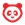cancel
Showing results for
Did you mean:

# How do I create a Numeric question in New Quizzes?

## How do I create a Numeric question in New Quizzes?

When creating an assessment, you can create a Numeric question in New Quizzes. Numeric questions will accept from students that contain numbers, text, and/or symbols. However, instructors can only use numeric entries for accepted answers. Student answers in non-numeric format will not be graded automatically.

You can also duplicate Numeric questions to create a new question.To create a question, click the Add button anywhere in the assessment.## Add Question Title and Stem

In the Question Title field , add a question title. Question titles are optional and are not visible to students.

In the Question Stem field , add the question stem. The question stem contains the question and, if necessary, additional instructions and information. You can use the Rich Content Editor to format and add content to your question stem.

## Select Requirement

To select a requirement for the answer, select an option from the Requirement drop-down menu . You can select Exact Response, Margin of Error, Within a Range, or Precise Response.

### Select Exact Response

To add an exact response answer, select the Exact Response option . Enter an answer by typing the answer in the Answer field  or using the arrows . Exact response answers can be added in scientific notation (accepted format: 1.234*10^5).

Students responses will be marked correct if they match the answer exactly.

Note: The Range fields only accept numbers, periods, commas, asterisks, and carets.

### Select Margin of Error

To add a margin of error answer, select the Margin of Error option . Enter the answer in the Answer field . Then add the margin in the Margin field  and select the margin type in the Margin Type drop-down menu . You can select percentage or absolute margin type. Margin of error fields do not accept entries written in scientific notation.

Student answers will be marked correct if they fall within the margin above or below the answer.

Note: The Answer and Margin fields only accept numbers, periods, and commas.

### Select Within a Range

To add an answer that must fall within a specific range, select the Within a Range option . Then select the range start  and range end for the answer . Range inputs can be in scientific notation (accepted format: 1.234*10^5).

Student responses will be marked correct if they are greater than or equal to the range start and less than or equal to the range end.

Note: The Range fields only accept numbers, periods, commas, asterisks, and carets.

### Select Precise Response

To add an answer with a precise requirement, select the Precise Response option . Then select the answer , precision value , and precision type . The options for precision type are significant digits and decimal places. Precise response fields do not accept entries written in scientific notation.

Student responses will be marked as correct if they match the answer to the given number of significant digits or decimal places.

Note: The Answer field only accept numbers, periods, and commas. The Precision field only accepts whole numbers greater than 1.

## Enable On-Screen CalculatorTo allow students to use an on-screen calculator for this question, click the Show on-screen calculator checkbox .

If you select this option, you can choose either a basic calculator  or scientific calculator . The basic calculator displays numbers and basic math functions (add, subtract, multiply, divide). The scientific calculator displays more options for advanced calculations.

Note: The on-screen calculator option is intended to be used while displaying one question at a time. If the assessment does not display one question at a time, students may be able to use the calculator for all questions.

## Align to Outcome

To align the question to an outcome, click anywhere in the Align to Outcomes section.

Note: After a question has been added to an item bank, it can only be edited in the item bank. You can still set unique point values and options in the assessment for an item bank question.

## Add Point Value and FeedbackTo customize the point value for the question, type the point value in the Points field  or use the arrows to increase or decrease the point value .

To leave feedback for students, click the Feedback icon .

## Save QuestionTo save the question, click the Done button.

Version history
Revision #:
12 of 12
Last update:
yesterday
Updated by: